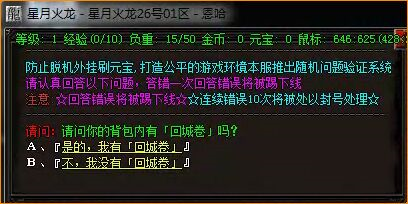# 七星辅助过回答问题防脱机验证脚本分享最近比较火的一个单职业gom引擎火龙版本，很多用户都在吐槽这个版本的验证码，现在七星辅助就来为大家详细的解答下，验证如上图所示。这个没有难度，就是根据实际情况来回答问题，唯一的难点就是问题有十几个给大家收集了一点，可以参照七星辅助过回答问题验证脚本如下：

<回收物品>

/<
a = 获取NPC对话内容()
if string.find(a, "请问你的背包内有「回城卷」吗？")~=nil then

end
if string.find(a, "请问你是否已经加入了行会？")~=nil then

end
if string.find(a, "请问你是什么职业？")~=nil then

end
if string.find(a, "所在地图是否是安全区呢？")~=nil then

end
if string.find(a, "请问你的背包内有「盟重传送石」吗？")~=nil then

end
if string.find(a, "请问你拥有技能「灵魂火符」吗？")~=nil then

end
if string.find(a, "请问你拥有技能「火墙」吗？")~=nil then

end
if string.find(a, "请问你包内有修复神水吗？")~=nil then

end
if string.find(a, "请问你的背包内有「金条」吗？")~=nil then

end
if string.find(a, "请问你拥有技能「狮子吼」吗？")~=nil then

end
if string.find(a, "您在游戏中的人物角色是男性还是女性？")~=nil then

end
if string.find(a, "请问你拥有技能「魔法盾」吗？")~=nil then

end
if string.find(a, "您是否已经加入了本服的会员呢？")~=nil then

end
if string.find(a, "请问你的等级达到80级了吗？")~=nil then

end
if string.find(a, "请问你包内有疗伤圣水吗？")~=nil then

end
if string.find(a, "请问你拥有技能「地狱雷光」吗？")~=nil then

end
if string.find(a, "请问你拥有技能「烈火剑法」吗？")~=nil then

end
if string.find(a, "请问你的背包内有50万元宝吗？")~=nil then

end
if string.find(a, "请问你拥有技能「隐身术」吗？")~=nil then

end
/>

更多的验证码脚本分享，大家可以参考七星辅助官网。问题答案该修改的就修改下，没有的问题自己添加下。

85%的户主都看了以下辅助验证码下载